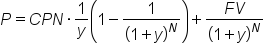+

# Valuing Bonds

Author: Sophia Tutorial
##### Description:

Determine the market value of a bond based on its Yield to Maturity.

(more)### Developing Effective Teams

*No strings attached. This college course is 100% free and is worth 1 semester credit.

46 Sophia partners guarantee credit transfer.

299 Institutions have accepted or given pre-approval for credit transfer.

* The American Council on Education's College Credit Recommendation Service (ACE Credit®) has evaluated and recommended college credit for 33 of Sophia’s online courses. Many different colleges and universities consider ACE CREDIT recommendations in determining the applicability to their course and degree programs.

Tutorial

what's covered
In this lesson, you will learn about the market value of a bond based on its yield to maturity. Specifically, this lesson will cover:
1. Valuing Bonds
1. At Par
2. At a Discount

## 1. Valuing Bonds

As with all financial securities, the true value of a bond can be calculated from the net present value of all the expected cash flows. If a bondholder holds a bond to maturity, they will receive interim interest payments at the coupon rate and the face value at maturity.

big idea
The value of a bond is the present value of each of these cash flows added together.

Consider the cash flows on a timeline. If we are going to receive these coupon payments and the face value at maturity, what is the interest rate at which the present value equals the price of the bond today?

hint
Price can be quoted in dollars, but it is often quoted as a percent of face value. For example, if a bond with a \$1000 face value is selling for \$980, it can be said that the bond is selling at 98%. If it was selling at \$1050, it would be said that the bond is selling at 105%.

We can determine this by directly solving the following equation:

formula

Yield to Maturity of a Coupon BondIn this formula, the variables are equivalent to:
• P: price today
• CPN: coupon payment
• FV: face value at maturity
• y: interest rate
Solving for the interest rate can prove quite complicated, but it is important, because this rate is called the yield to maturity. It is like the internal rate of return for the bond cash flows over the market price. Fortunately, there are tools for this, including spreadsheet software like Excel and other web applications. Let's take a look.

2a. At Par
Suppose a bond has a face value of \$1,000, with a coupon rate of 8%, and five years left to maturity. The coupon payments are semi-annual and the bond is currently trading at \$1,000, meaning it is trading at par. What is the yield of maturity?

 Face Value: \$1,000 Annual Coupon Rate: 8% Years to Maturity: 5 Coupon Payments per Year: 2 Current Bond Price: \$1,000 Yield to Maturity: 8.00%

The yield to maturity, or the internal rate of return, would be 8%, which is the same as the coupon rate. This is due to the fact that the bond is currently selling at par.

term to know

Selling At Par
A bond’s coupon rate is equal to its yield to maturity (YTM).
2b. At a Discount
Suppose a bond has the same information as above, but now the bond is currently selling at \$940. What is the yield of maturity? Do you think it will be 8%, higher than 8%, or lower than 8%?

 Face Value: \$1,000 Annual Coupon Rate: 8% Years to Maturity: 5 Coupon Payments per Year: 2 Current Bond Price: \$940 Yield to Maturity: 9.54%

The yield to maturity would actually be 9.54%. It is higher than the coupon rate because the current price is low at \$940. If we are going to still get the face value at maturity in the same coupon payments, the net present value of this bond is worth more to us. We can say that this bond is selling at a discount.

term to know

Selling at a Discount
A bond’s coupon rate is less than its yield to maturity (YTM).
Suppose we have the same bond as above, but the price today is \$1,090. The bond is selling at a premium. What is the yield of maturity?

 Face Value: \$1,000 Annual Coupon Rate: 8% Years to Maturity: 5 Coupon Payments per Year: 2 Current Bond Price: \$1,090 Yield to Maturity: 5.90%

The yield to maturity would actually be 5.90%. It is lower than the coupon rate because the the bond is selling at a premium. Since we have to pay so much for it, the yield of maturity will be less.

term to know

A bond’s coupon rate is more than its yield to maturity (YTM).
summary
In this lesson, we learned about valuing bonds. Bonds pay a coupon payment periodically throughout their life to maturity and most often this interest rate is fixed. At maturity, the holder receives the face value, which is also fixed. With these two components being fixed, what changes in the market is the price. The interest rate at which the discounted cash flows of the coupon and maturity payments equal the price is the yield to maturity.

We also learned how to compare the yield of maturity for bonds that are currently at par, at a discount, and at a premium.

Best of luck in your learning!

Source: THIS CONTENT HAS BEEN ADAPTED FROM LUMEN LEARNING'S “Valuing Bonds” TUTORIAL.

Terms to Know
Selling At Par

A bond’s coupon rate is equal to its yield to maturity (YTM).

Selling at a Discount

A bond’s coupon rate is less than its yield to maturity (YTM).